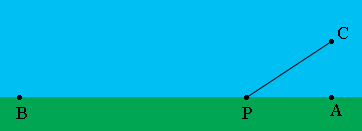SEARCH HOMEMath Central Quandaries & QueriesQuestion from Christy, a student: Hello, I have no idea where to start with this question. Bob is at point B, 35 miles from A. Alice is in a boat in the sea at point C, 3 miles from the beach. Alice rows at 2 miles per hour and walks at 4.25 miles per hour, where along the beach should she land so that she may get to Bob in the least amount of time?Hi Christy,

I think the problem should say "Alice is in a boat in the sea at point C, 3 miles from the closest point A on the beach." here is my diagramAlice is to row from C to some point P on the beach and then walk along the beach to Bob.

Put a coordinate system on the diagram with the origin at B and with the shoreline being the X-axis. C has coordinates (35, 3). Let P have coordinates (x, 0). Use the distance formula to write an expression for the distance from C to P. How long does it take Alice to row from C to P? How long does it take her to walk from P to B? What is her total time? Use the calculus you know to find the value of x that minimizes this time.

PennyMath Central is supported by the University of Regina and The Pacific Institute for the Mathematical Sciences.